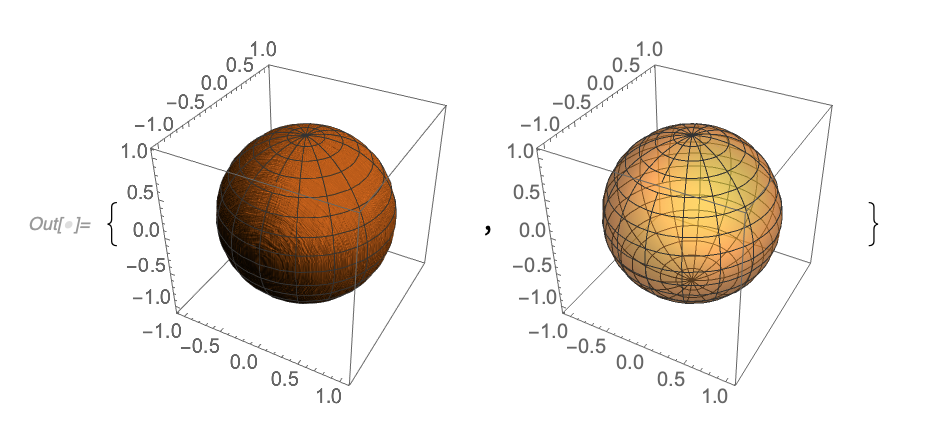I attempted to modify a plot for an electric field solution that I had in my old Antenna-Theory notes:
\BE
=
j \omega
\frac{\mu_0 I_{\textrm{eo}} l}{4 \pi r} e^{-j k r}
\lr{ 1 + \cos\theta }
\lr{
-\cos\phi \thetacap
+ \sin\phi \phicap
},
\end{equation}
and discovered that you can crash Mathematica (12.1.0.0) by combining PlotStyle with Opacity and HatchShading (new in 12.1).  Here’s a stripped down version of the plot code that demonstrates the crash:

ClearAll[ rcap]
rcap = {Sin[#1] Cos[#2], Sin[#1] Sin[#2], Cos[#1]} & ;

{
ParametricPlot3D[
rcap[t, p]
, {t, 0, π}
, {p, 0, 2 π}
, PlotStyle -> { HatchShading[0.5, Black]}
]
, ParametricPlot3D[
rcap[t, p]
, {t, 0, π}
, {p, 0, 2 π}
, PlotStyle -> {Directive[Opacity[0.5]]}
], ParametricPlot3D[
rcap[t,p]
,{t,0,π}
,{p,0,2 π}
Black]}
]
}

The first two plots, using one, but not both of, Opacity or HatchShading work fine:In this reproducer, the little dimple at the base has been removed, which was the reason for the Opacity.

I’ve reported the bug to Wolfram, but wonder if they are going to come back to me saying, “Well, don’t do that!”

EDIT: Fixed in Mathematica 12.1.1